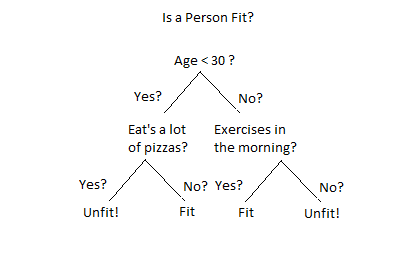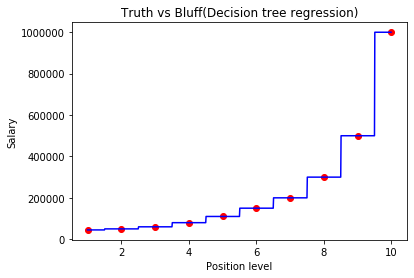×

Search anything:

# Decision Trees using Scikit-learn

#### Machine Learning (ML)Reading time: 20 minutes | Coding time: 10 minutes

In this article, we will understand decision tree by implementing an example in Python using the Sklearn package (Scikit Learn).

Let's first discuss what is a decision tree.
A decision tree has two components, one is the root and other is branches. The root represents the problem statement and the branches represent the solutions or consequences.Initially the problem or the root is split into two branches or consequences, and from the branches again a split occurs and further branches are created.

Regression trees- When the decision tree has a continuous target variable. For example, a regression tree would be used for the price of a newly launched product because price can be anything depending on various constraints.

Consider this example for decison tree!In this example we decide if a person is fit or not. And a person's fitness depends on various constraints.

Now let us build a decision tree regression model.

``````#importing the libraries
import numpy as np
import pandas as pd
import matplotlib.pyplot as plt
%matplotlib inline
``````

Importing the libraries numpy for linear algebra matrices, pandas for dataframe manipulation and matplotlib for plotting and we have written %matplotlib inline to view the plots in the jupyter notebook itself.

``````#importing the dataset
``````

Suppose we want to predict the salary for a new employee whose level of experience is 6.5 and he said that the previous company paid him 160000 and he wants a higher salary and we have got some data which has three columns- Position,Level and salary. then here we will use decision tree to predict his salary based on the data we have.

``````dataset.info
``````

now we divide our dataset into X and y, where X is the independent variable and y is the dependent variable.

``````X=dataset.iloc[:,1:2].values
y=dataset.iloc[:,2].values
``````
``````#fitting the decision tree regression model to the dataset
from sklearn.tree import DecisionTreeRegressor
regressor=DecisionTreeRegressor(random_state=0)
regressor.fit(X,y)
``````

we are training the entire dataset here and we will test it on any random value. Suppose the new employee said he has a experience of 6.5 years so we will predict his salary based on that.

``````y_pred = regressor.predict([[6.5]])
``````

Now let's check what is the predicted salary for the new employee.

``````y_pred
``````

It returns 150000. which is a little less than the salary that the employee told us.
Now visualising the results

``````#visualising the decision tree regression result(for higher resolution and smoother curves)
X_grid=np.arange(min(X),max(X),0.01)
X_grid.shape
``````
``````#reshaping X_grid from 1-D array to 2-D array
X_grid=X_grid.reshape(len(X_grid),1)
``````
``````plt.scatter(X,y,color='red')
plt.plot(X_grid,regressor.predict(X_grid),color='blue')
plt.title('Truth vs Bluff(Decision tree regression)')
plt.xlabel('Position level')
plt.ylabel('Salary')
plt.show()
``````

The graph will look like thisSo, we can conclude that the prediction is not accurate but close to the real value.
To overcome this accuracy issue we have another algorithm called Random forest.Random forest is an ensemble learning algorithm, which uses multiple algorithms together to get the result.

Random forest uses many decision trees so, it makes our predictions more accurate.